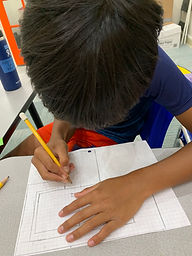Ms. Kayla

Target 1​

Lesson Type:

Continuation

Measurment

:

Techniques and Formulas

Solve problems involving area.

1:

Recognize area as a measurement of 2D shapes.

2:

Recognize that area is measured in squared units (i.e., cm2), depicting that the measurement has both height and width.

3:

Find the area of composite figures.

4:

Decompose a composite figure into simple rectangles to compute area.

5:

Use the formula for finding the area of rectangular shapes (A = base x height).

4th

Vocabulary:

Area, Squared units, Dimensions, Base/Length, Height

Activities:

1. Students started with creating a floor plan for a house with different room dimensions.

2. Students then broke their floor plans into different "rooms" and found area for each "room".

3. Then students found the area of their entire house in square units.Home Exploration

Guiding Questions:Absent Students:

Target 2

:

1:

Understand that there are multiple ways to divide multi-digit numbers.

Vocabulary:

Division, Multiplication, Skip Count, Dividend, Divisor, Quotient

Activities:

1. Students took what they know about multiplication to divide math facts (inverse). Students used multiple strategies to find the quotientanswer (). such as skip counting, multiplication, etcHome Exploration

Guiding Questions:Target 3

:

Vocabulary:

Activities:Home Exploration